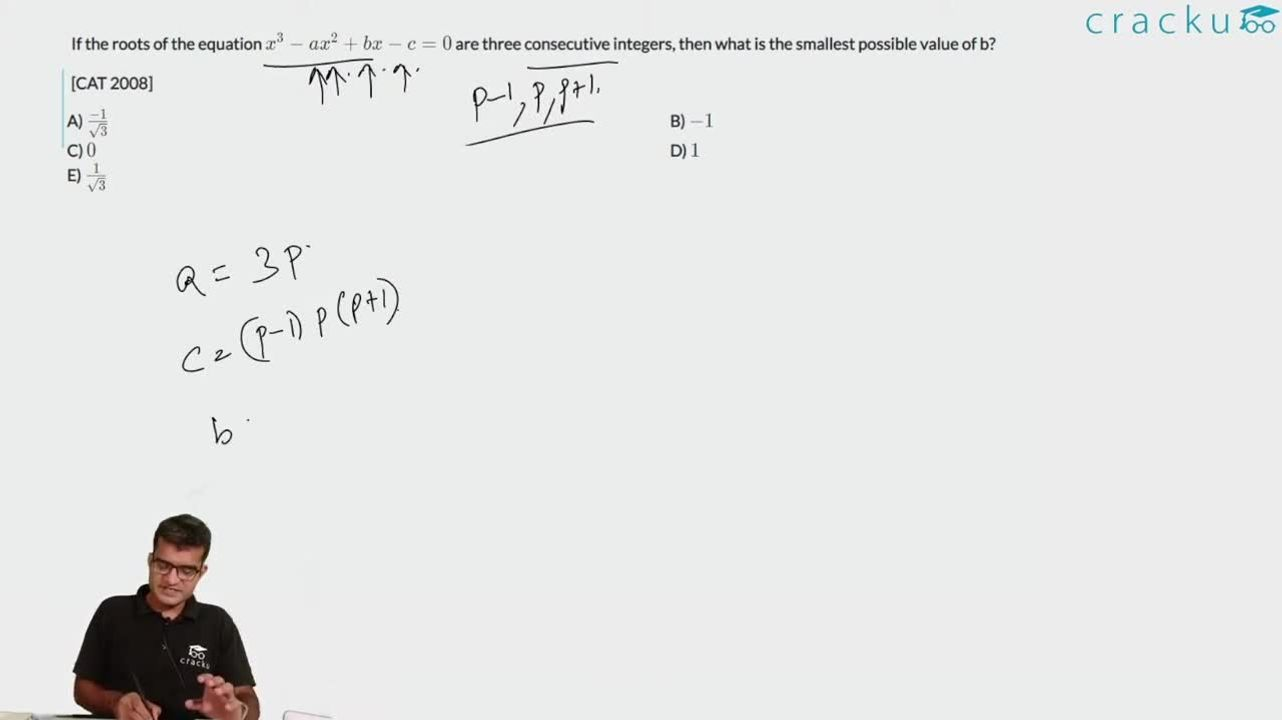Question 5

# If the roots of the equation $$x^3 - ax^2 + bx - c = 0$$ are three consecutive integers, then what is the smallest possible value of b?[CAT 2008]

Solution

b = sum of the roots taken 2 at a time.
Let the roots be n-1, n and n+1.
Therefore, $$b = (n-1)n + n(n+1) + (n+1)(n-1) = n^2 - n + n^2 + n + n^2 - 1$$
$$b = 3n^2 - 1$$. The smallest value is -1.

### View Video Solution